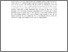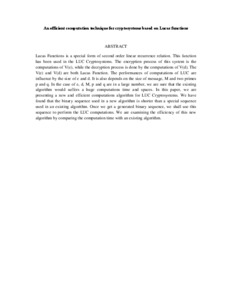# An efficient computation technique for cryptosystems based on Lucas functions

## Citation

Md Ali, Zulkarnain and Othman, Mohamed and Md. Said, Mohamad Rushdan and Sulaiman, Md. Nasir (2008) An efficient computation technique for cryptosystems based on Lucas functions. In: International Conference on Computer and Communication Engineering 2008 (ICCCE08), 13-15 May 2008, Kuala Lumpur, Malaysia. (pp. 187-190).

## Abstract

Lucas Functions is a special form of second order linear recurrence relation. This function has been used in the LUC Cryptosystems. The encryption process of this system is the computations of V(e), while the decryption process is done by the computations of V(d). The V(e) and V(d) are both Lucas Function. The performances of computations of LUC are influence by the size of e and d. It is also depends on the size of message, M and two primes p and q. In the case of e, d, M, p and q are in a large number, we are sure that the existing algorithm would suffers a huge computations time and spaces. In this paper, we are presenting a new and efficient computations algorithm for LUC Cryptosystems. We have found that the binary sequence used in a new algorithm is shorter than a special sequence used in an existing algorithm. Once we get a generated binary sequence, we shall use this sequence to perform the LUC computations. We are examining the efficiency of this new algorithm by comparing the computation time with an existing algorithm.Preview
Text (Abstract)
An efficient computation technique for cryptosystems based on Lucas functions.pdfView Item